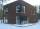# Arithmetic progression + divisibility - math problems

#### Number of problems found: 6

• Real estateResidential house has three entrances numbered by odd numbers arithmetic progression. The sum of the two numbers on the corner entrances is 50. Calculate the highest of this three numbers.
• CalculateCalculate the sum of all three-digit natural numbers divisible by five.
• Sum of odd numbersFind the sum of all odd integers from 13 to 781.
• Have solutionThe sum of four consecutive even numbers is 92. Determine these numbers.
• The sum 2The sum of five consecutive even integers is 150. Find the largest of the five integers. A.28 B.30 C.34 D.54 Show your solution and explain your answer.
• Odd numbersThe sum of four consecutive odd numbers is 1048. Find those numbers ...

We apologize, but in this category are not a lot of examples.
Do you have an interesting mathematical word problem that you can't solve it? Submit a math problem, and we can try to solve it.

We will send a solution to your e-mail address. Solved examples are also published here. Please enter the e-mail correctly and check whether you don't have a full mailbox.

Please do not submit problems from current active competitions such as Mathematical Olympiad, correspondence seminars etc...

Arithmetic progression - math word problems. Divisibility - math word problems.